# Chapter 2 How to Visualize a Single Variable

## 2.1 Get to know the dataset

When opening a dataset, we don’t always know what we are dealing with. Some data can be so large it’s hard to tell how many variables and how many cases there are. Before zooming into a single variable, it’s always a good idea to get to know the data a little bit. Here’s an example with the titanic data:

Opening the data (don’t forget to set working directory first)

library(foreign)
attach(titan)

Take a look at the dataset in a new tab

View(titan)

Figure out how many cases and variables there are in this dataset

dim(titan)
##  2201    4

The first number is the # of cases, the second number is # of variables

Take a look at the first 6 rows (cases) of the dataset:

head(titan)
##   Class    Sex   Age Survived
## 1  3rd  Female Child        N
## 2  3rd  Female Child        N
## 3  3rd  Female Child        N
## 4  3rd  Female Child        N
## 5  3rd  Female Child        N
## 6  3rd  Female Child        N

This allows us to get a quick sense of which variables are categorical and which are quantitative. Based on the above output, for example, it’s easy to tell that none of the variables are represented in numbers.

To figure out if a variable is categorical or not, the best way is to look at all its values.

table(Class)
## Class
## 1st  2nd  3rd  Cre
##  325  285  706  885

The above output shows that the variable “Class” has 4 categories–1st, 2nd, 3rd, and Cre (Crew). The numbers under are the number of people in each category. So this variable is categorical.

When typing our code, we often need to know the exact spelling of a variable name. Here’s an easy way to find out.

names(titan)
##  "Class"    "Sex"      "Age"      "Survived"

## 2.2 Visualizing a Categorical Variable

A picture of a categorical variable should show how many cases there are in each category, aka its distribution.

The most commonly used plots for categorical variables are bar plots and pie charts. We can also simply represent them by tables, but they are less eye-catching.

### 2.2.1 Bar plot

Here’s how to create a simple bar plot for the variable ‘Survied’ in the titanic data. Note that we are not directly creating a bar plot from the variable. The bar plot has to be derived from a table which we called ‘t.’

t=table(Survived)
barplot(t)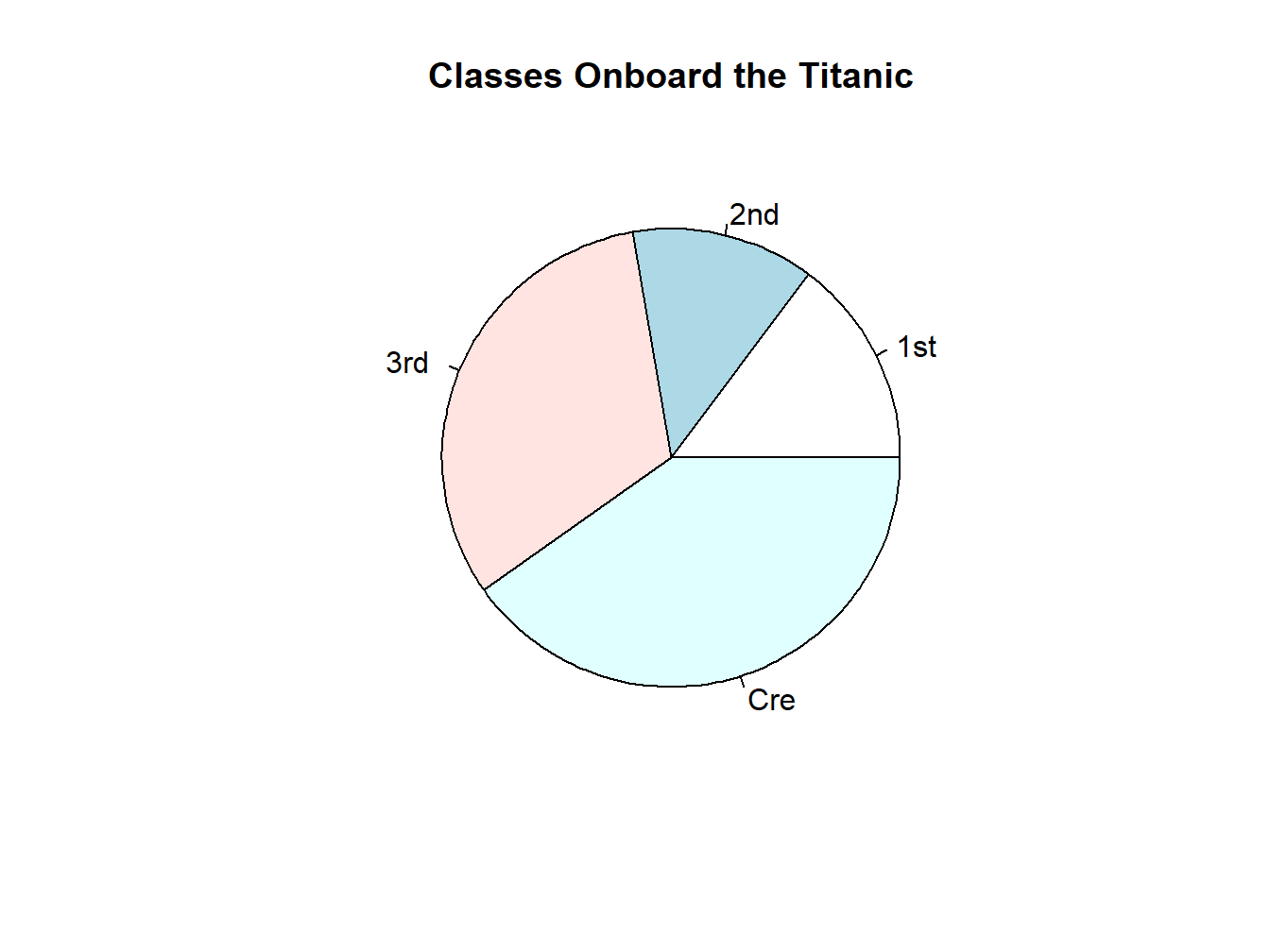Figure 2.1: An Unadorned Bar Plot

If you want to add a title to the bar plot:

barplot(t, main="Survival Onboard the Titanic")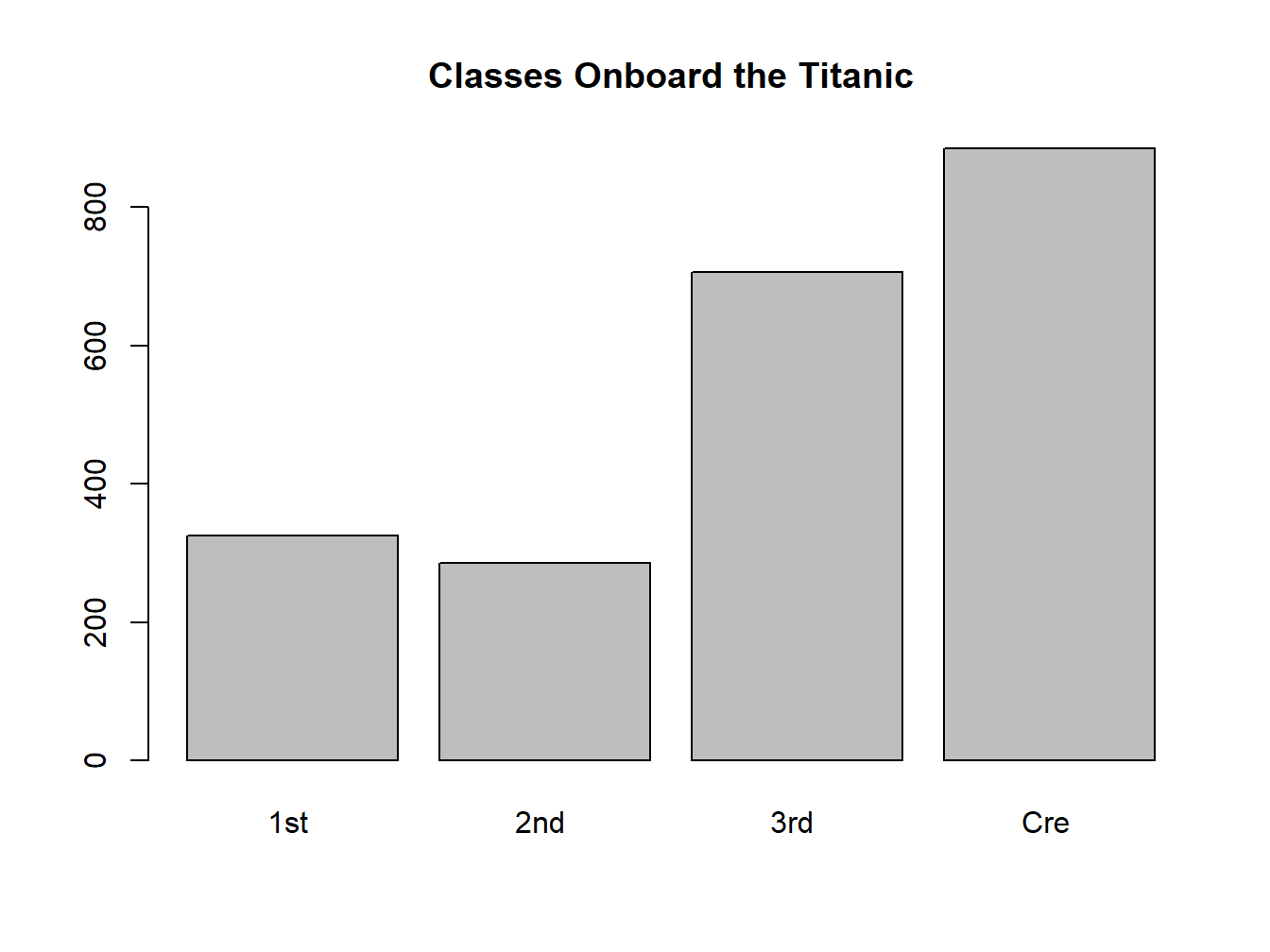Figure 2.2: A Titled Bar Plot

If you want to give it some color:

barplot(t, main="Survival Onboard the Titanic",col="blue")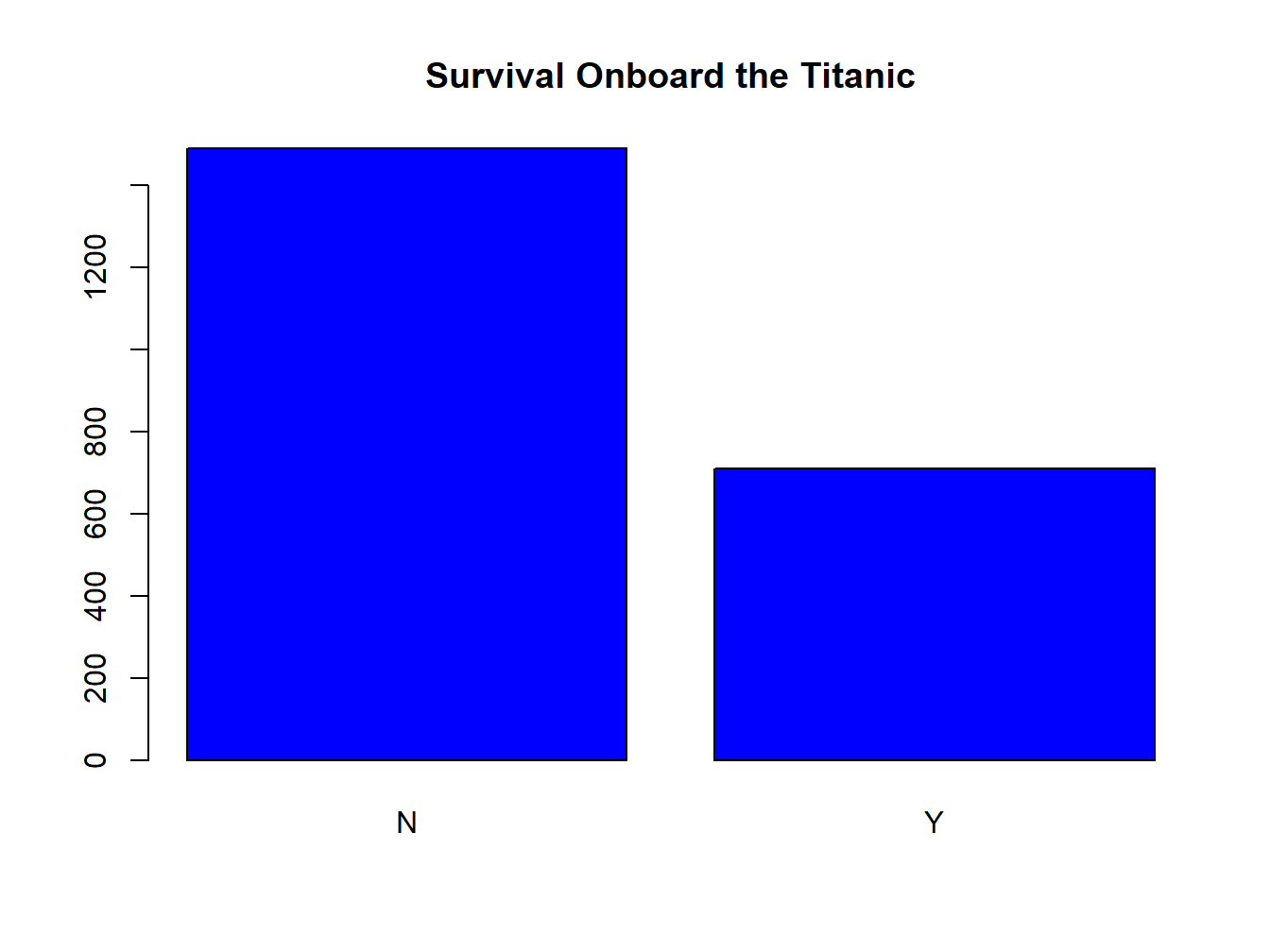Figure 2.3: A Colored Bar Plot

### 2.2.2 Pie Chart

Create a pie chart for variable ‘Survived.’ Note that I can directly use ‘t’ here because ‘t’ has been created earlier as a table of ‘Survived.’ If you haven’t created this table, you’d get an error message, since R doesn’t know what you are talking about.

pie(t)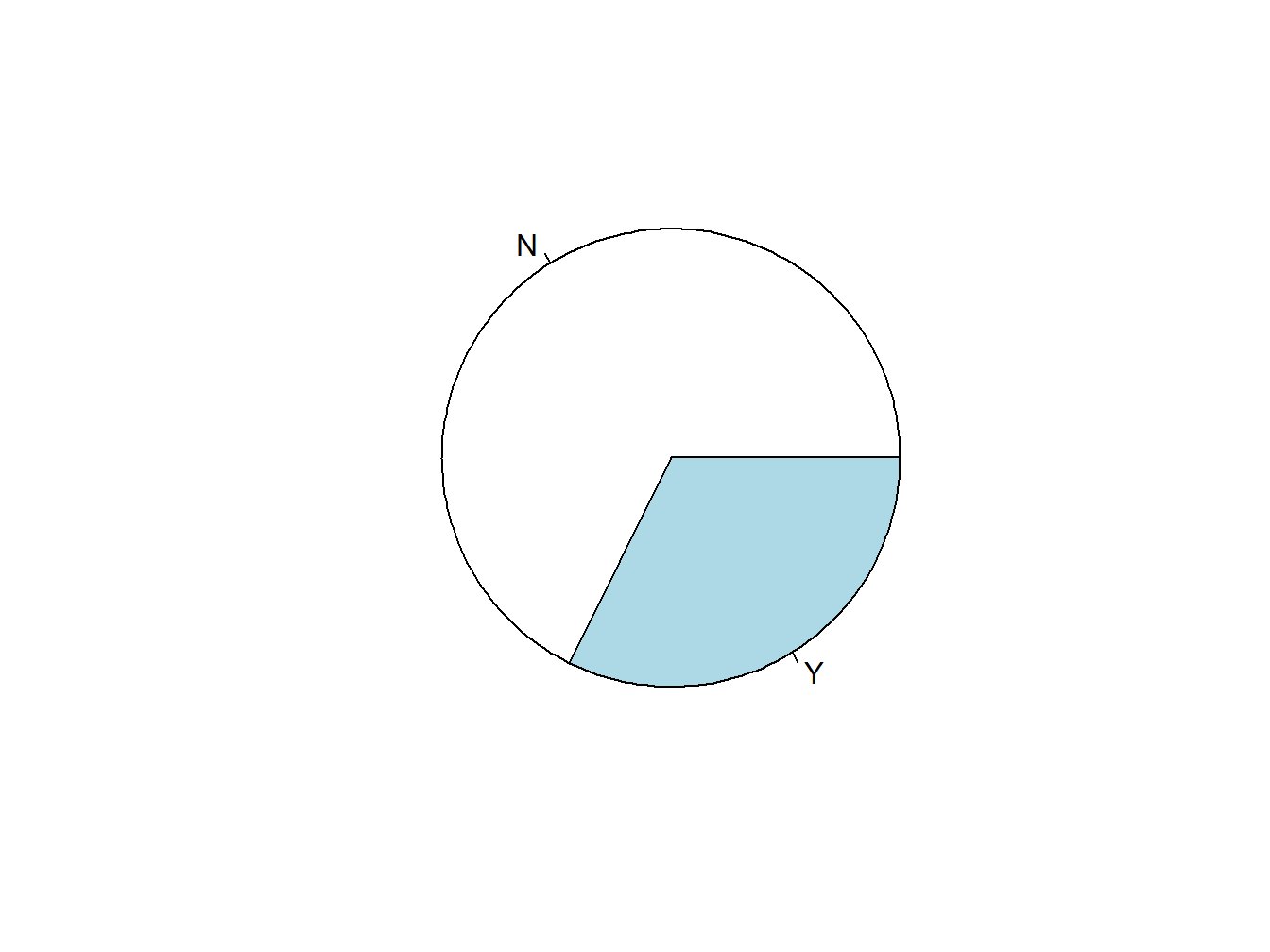Figure 2.4: An Unadorned Pie Chart

Add a title to the pie chart:

pie(t, main="Survival Onboard the Titanic")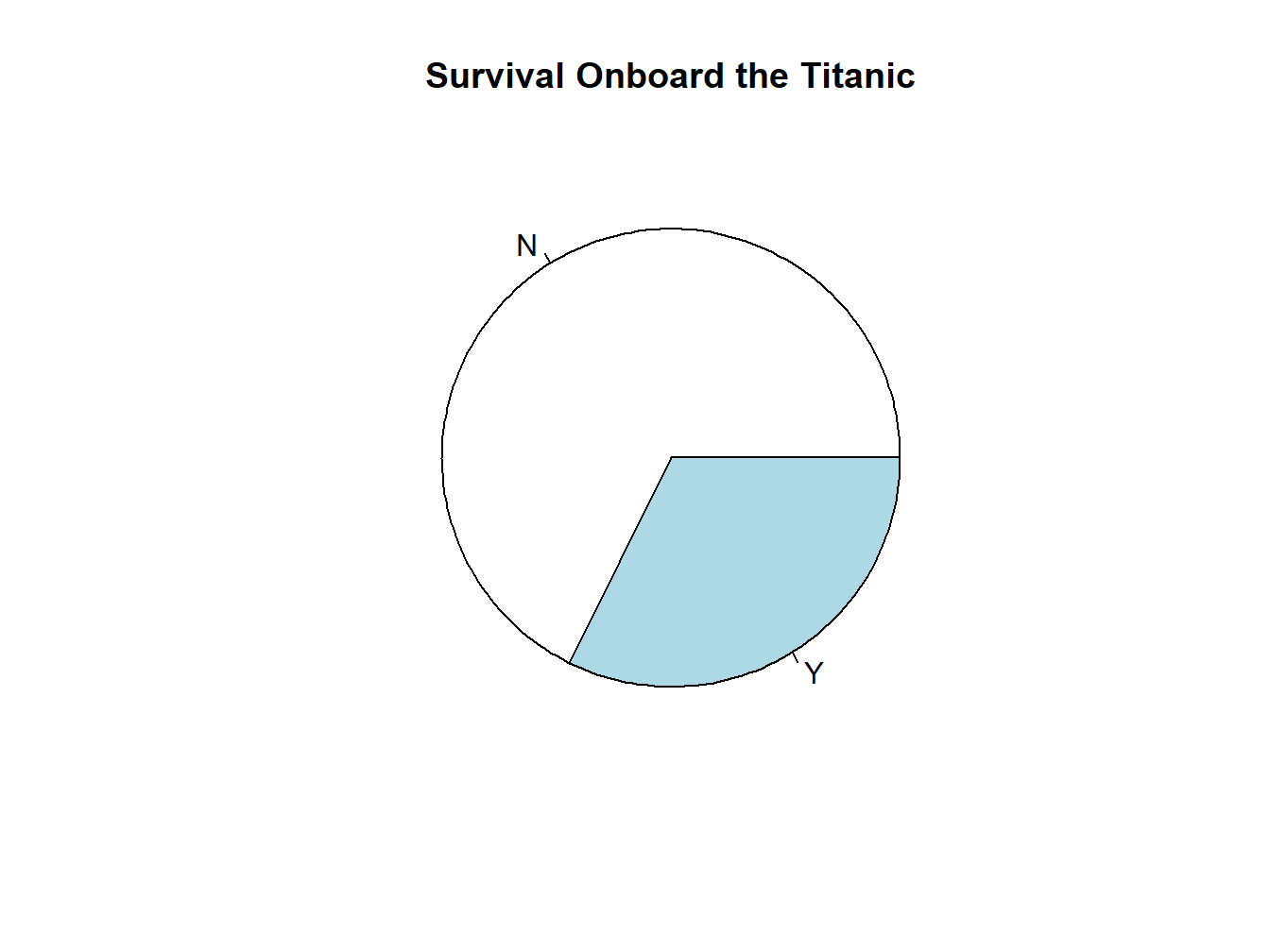Figure 2.5: A Titled Pie Chart

pie(t, main="Survival Onboard the Titanic",col=c("blue","red"))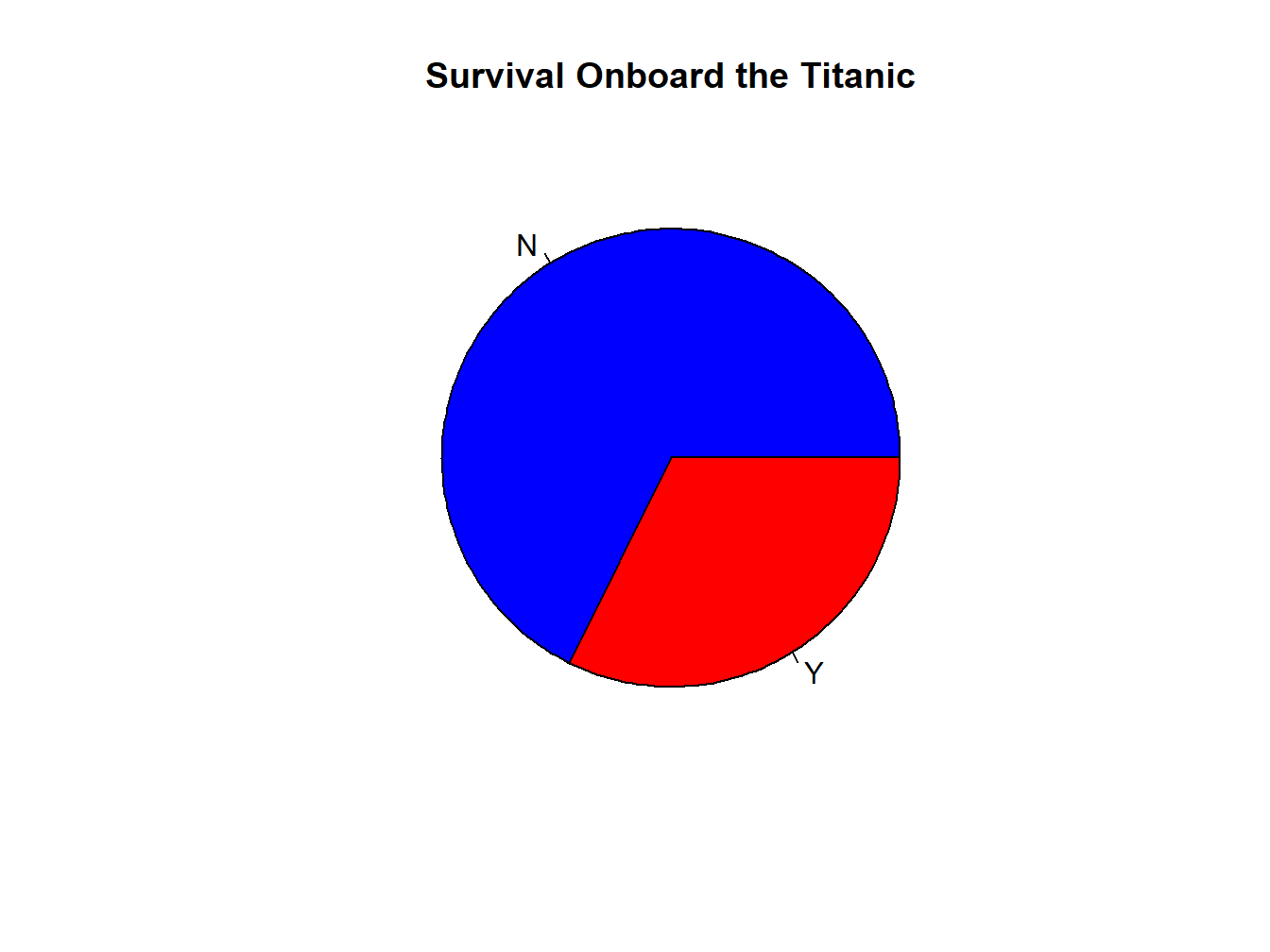Figure 2.6: A Colored Pie Chart

### 2.2.3 Bar or Pie?

This could be a question of personal taste, but here’s the rule of thumb that I use: when there are more than 4-5 categories in the variable, I’d use a bar plot rather than a pie chart.

For example, can you compare the numbers of people in each class in this pie chart? You probably can, but you can see how it gets increasingly difficult when there are more categories there.

t.class=table(Class)
pie(t.class, main="Classes Onboard the Titanic")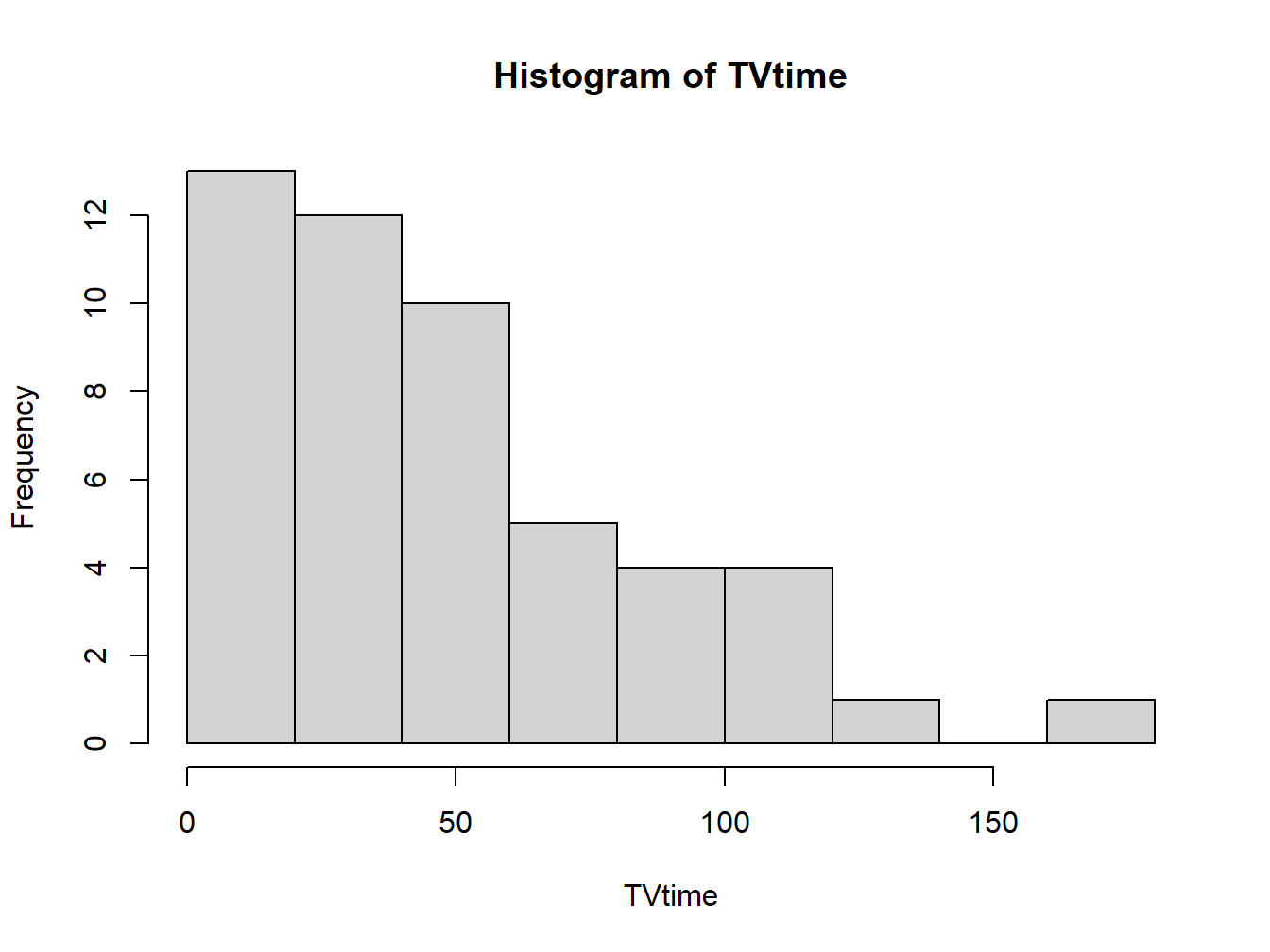Whereas a bar plot makes it a lot easier to compare:

barplot(t.class, main="Classes Onboard the Titanic")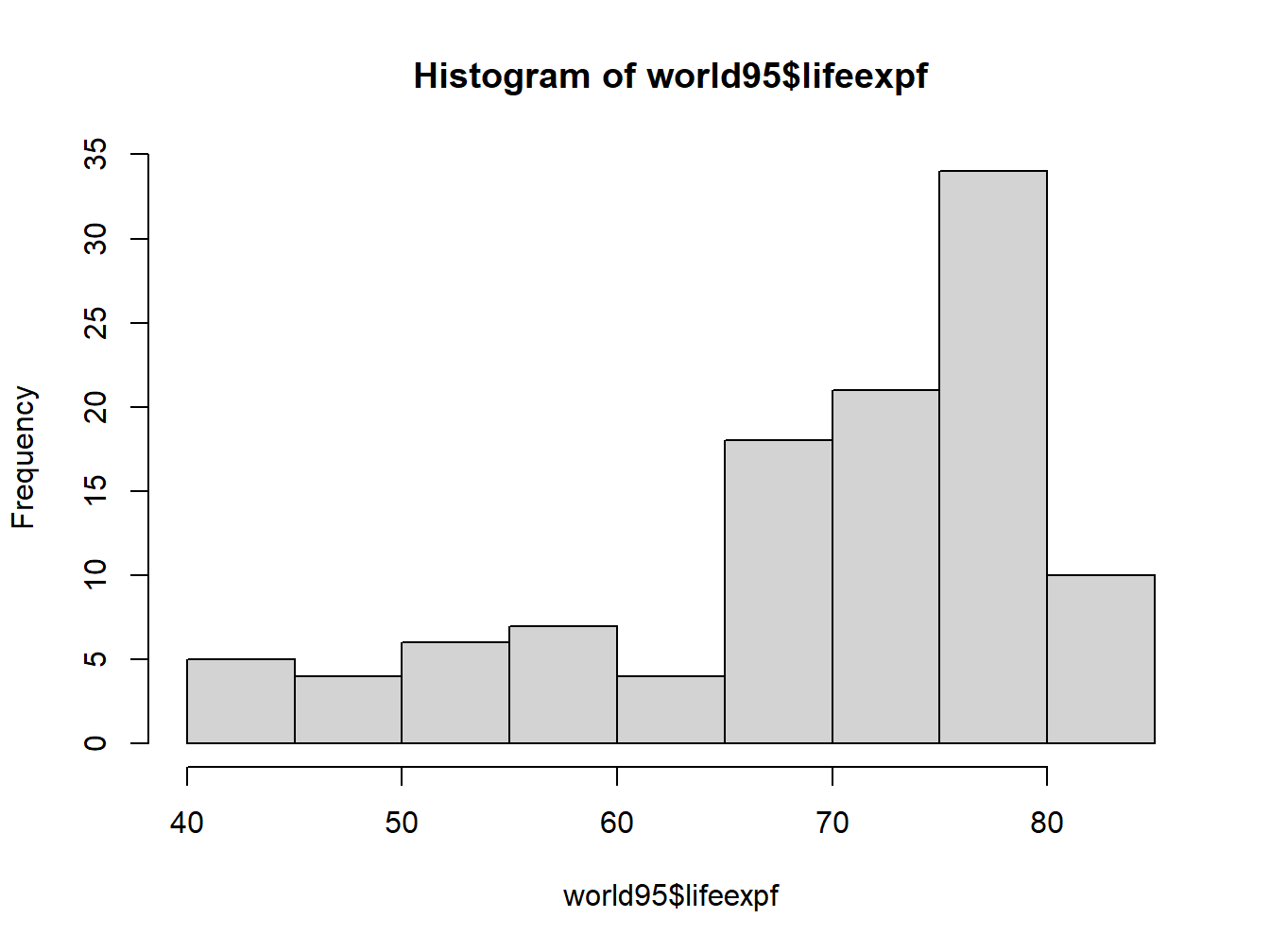Create a pie chart and a bar plot for Sex, Age, and Class in ‘Titanic.’

## 2.3 Visualizing a Quantitative Variable

A picture of a quantitative variable should show how many cases there are for each value, aka its distribution.

The most commonly used plots for quantitative variables are histograms and boxplots. We do NOT usually represent them by tables. Although creating a table for a variable of any sort will allow you to quickly judge if it is quantitative or not, it is not something that you want to include in a professional presentation if the variable happens to be quantitative–not readable!

Let us now load another dataset called ‘TV.sav.’ Again, don’t forget to set working directory first!

library(foreign)
attach(tv)

How many people and how many variables are there in this dataset?

dim(tv)
##  50  1

Are the variables categorical or quantitative?

head(tv)
##   TVtime
## 1     30
## 2     60
## 3     40
## 4     12
## 5     33
## 6     30

Take a careful look at the variable names so we can use them later:

names(tv)
##  "TVtime"

### 2.3.1 Histogram

Here’s how to create a histogram for the variable ‘NumPartner.’ Note that it’s simpler than creating a bar plot

hist(TVtime)Change the number of bars in the histogram:

hist(TVtime,breaks=50)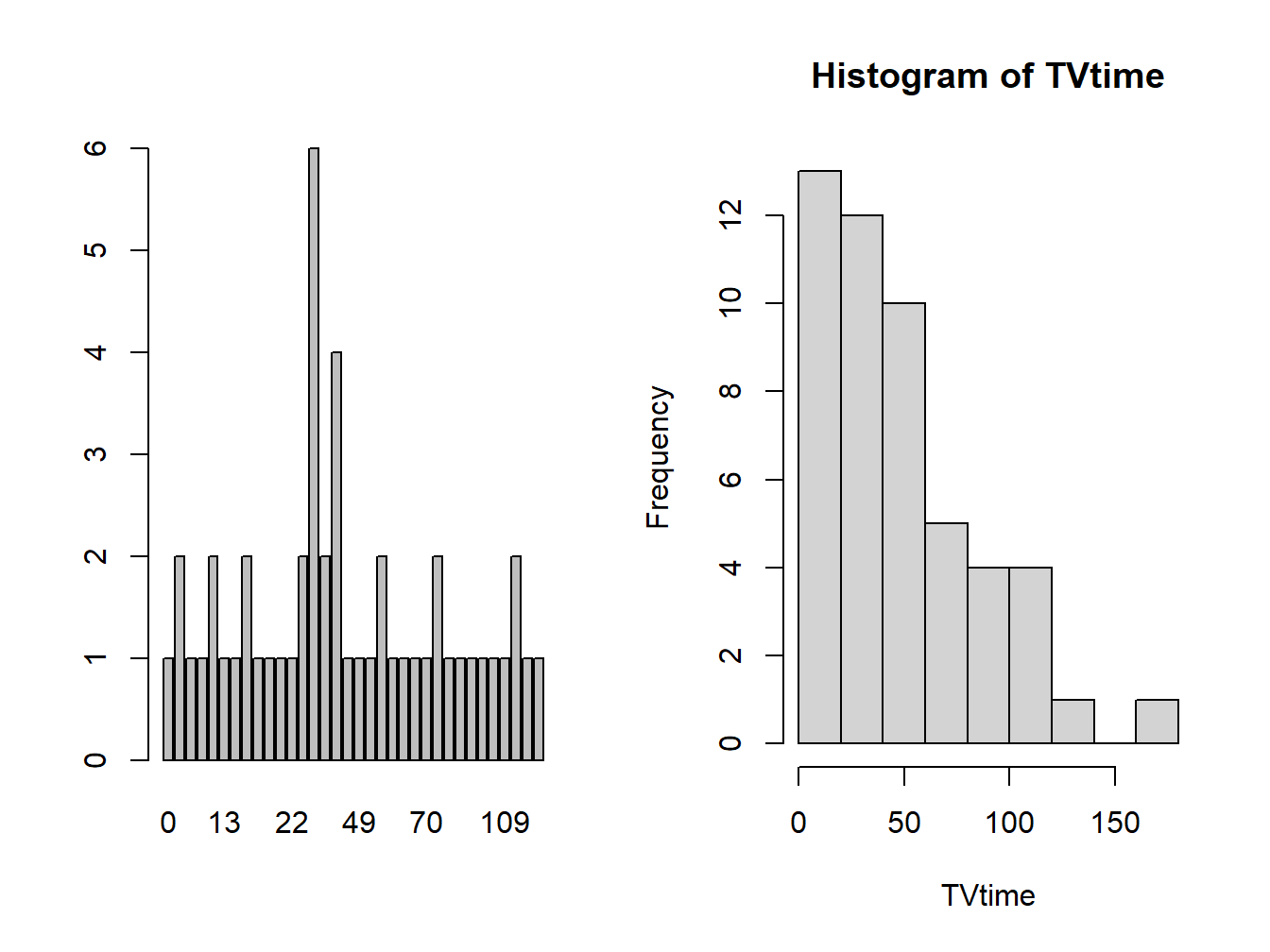Figure 2.8: Histogram with More Bars

Add some colors to the histogram:

hist(TVtime, col="red")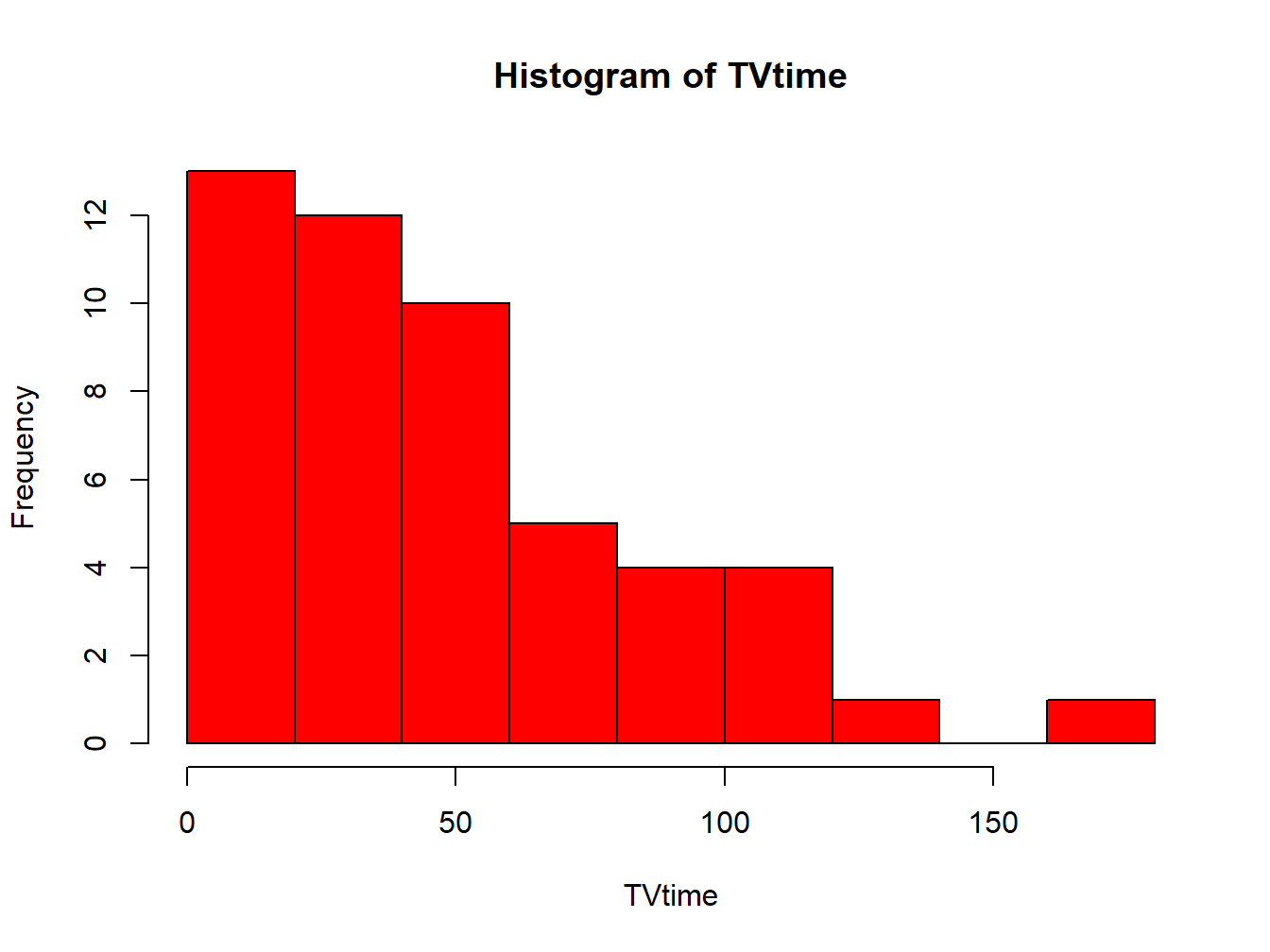Figure 2.9: A Red Histogram

hist(TVtime, col="lightblue", border="pink")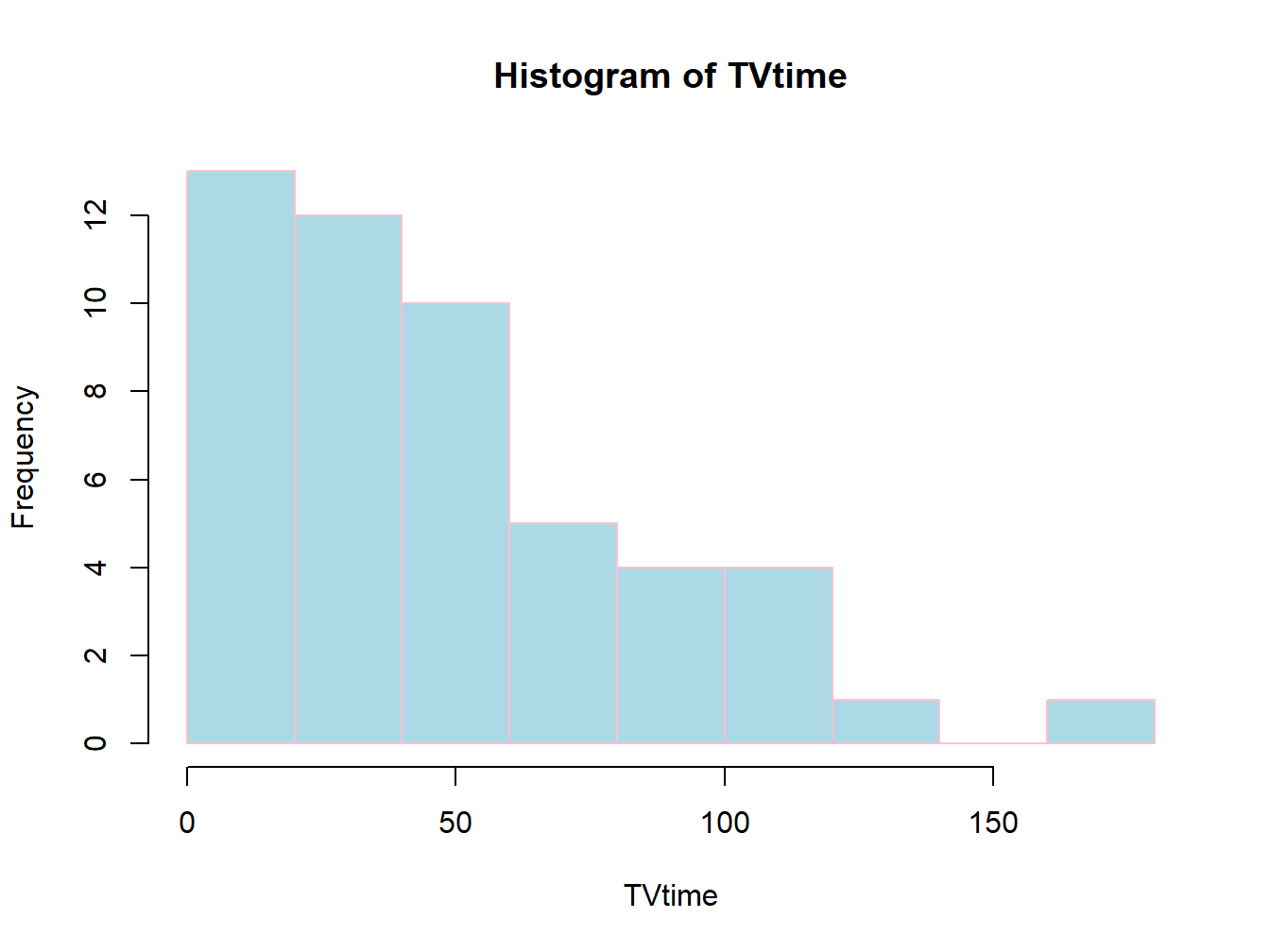Figure 2.10: Histogram with More Colors

hist(TVtime, main="Children's TV Watching Time in Minutes")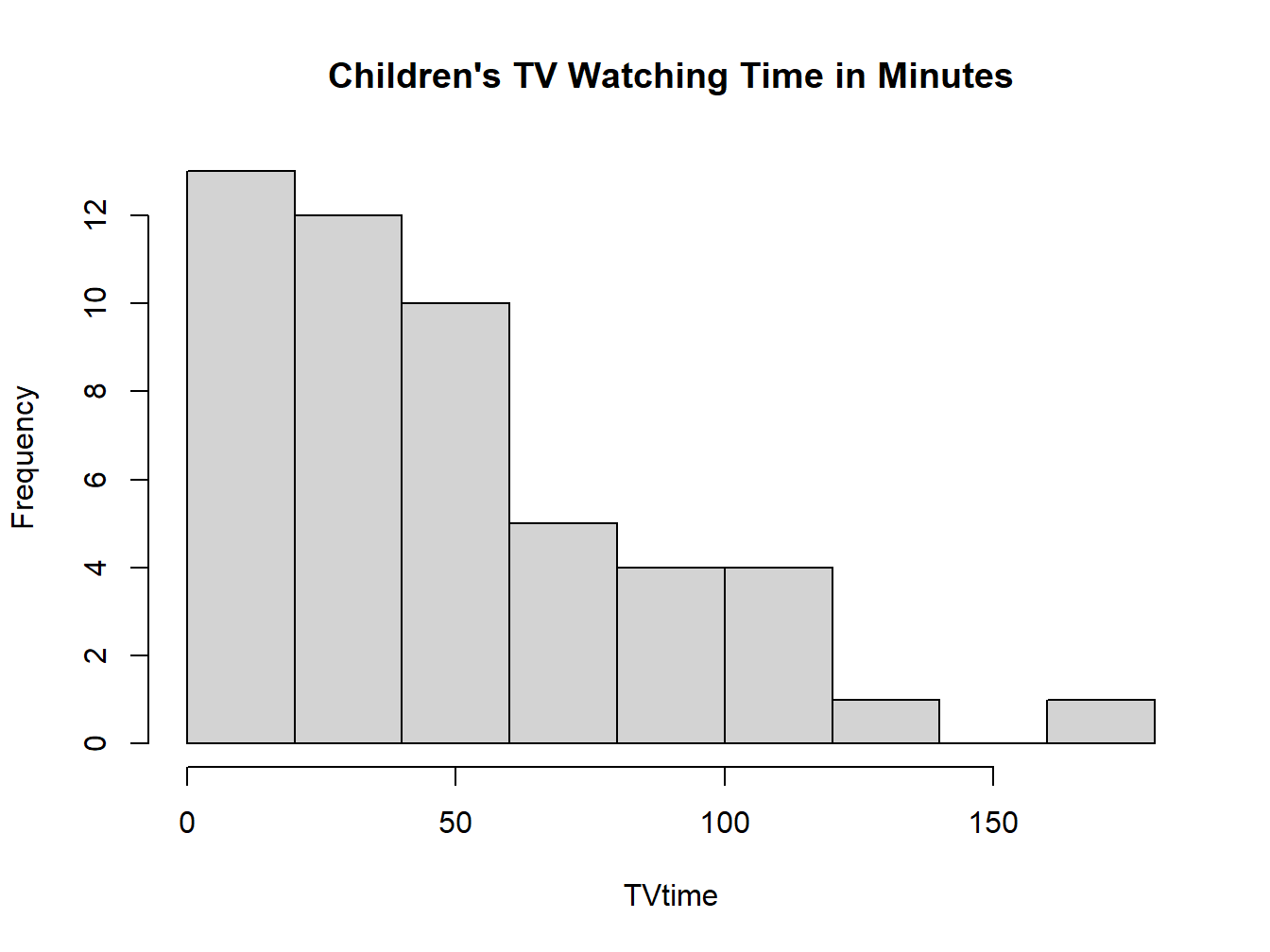Figure 2.11: Histogram with a Title

### 2.3.2 Boxplot

Boxplot is also known as box-and-whisker plot.

It is sometimes represented vertically, sometimes horizontally.

We will explain the boxplot in more details next week. For now, notice how the boxplot corresponds to the histogram.

Creating a boxplot is quite simple

boxplot(TVtime)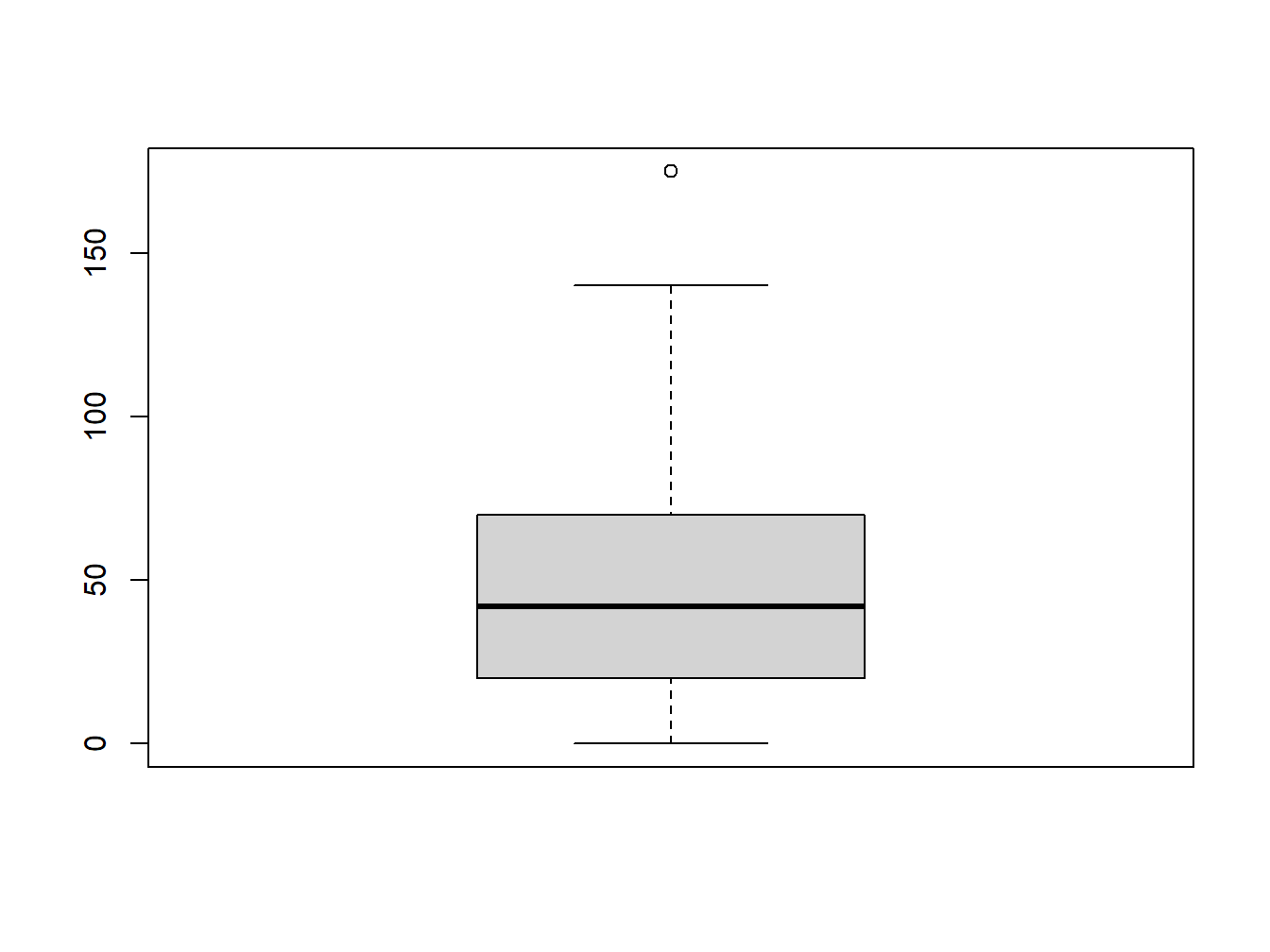Figure 2.12: Histogram with a Title

So, it looks like that if a variable has a long tail to the right in the histogram, its boxplot will have a long whisker on top.

If the variable has a long tail to the left in the histogram, can you picture what the boxplot would look like?

What if the variable has equal-length tails on both sides in the histogram?

### 2.3.3 Comparing a Histogram with a Bar Plot

Let’s create a bar plot for “TVtime”

t.tv=table(TVtime)
barplot(t.tv)

Put the bar plot and the hisogram side by side:

par(mfrow=c(1,2))
barplot(t.tv)
hist(TVtime)Figure 2.13: Histogram with a Title

What’s the difference between a bar plot and a histogram?

Why do we prefer the histogram to the bar plot when plotting quantitative variables?

Open and attach the data “chpt5.heights.sav”

1. How many people and how many variables are there in this dataset?

2. What are the variables?

3. Are they categorical or quantitative?

4. Create a histogram for the variable “Height,” add a title.

5. Create a boxplot for the variable “Height,” add a title

6. Say something about the hights of these people. On average are they tall or short? Are there more tall people than short people, or the other way around, or are there as many tall people as short people?

## 2.4 Something Else about Categorical Variables–Risk and Change of Risk

In the context of a categorical variable, we often talk about the risk or chance of doing something, such as:

• the risk of getting colon cancer
• the risk of dropping out of high school
• the chance of going to college (Note that the ‘risk’ of going to college doesn’t sound quite right.)

People often talk about risk/chance and increased/decreased risk/chance without really knowing what they mean. Here are some examples to help you understand:

### 2.4.1 Example 1

Variable: getting colon cancer or not. We’ll name the event of getting colon cancer as ‘C.’

The risk of getting colon cancer, i.e. the probability of getting colon cancer would be $$P(C)$$.

$P(C)=\frac{\mbox{# of people with colon cancer in our data}}{\mbox{Total # of people in our data}}$

Whenever we talk about the ‘increased risk’ or ‘decreased risk’ of something, it means that a second variable is taken into account, e.g.

1st Variable: getting colon cancer or not.

2nd Variable: eat bacon or not.

In the news, you sometimes hear them say “If you eat bacon regularly, your chance of getting colon cancer is increased by 18%.” This number, 18%, is the increased risk of bacon-eaters over non-bacon-eaters in terms of getting cancer. What does it really mean?

$\mbox{Increased Risk of C: Bacon v. No Bacon}=\frac{P(C)_{Bacon}-P(C)_{No Bacon}}{P(C)_{No Bacon}}$ The numerator is easy to understand. It’s just the difference between bacon eaters and non-bacon eaters in their chance of getting colon cancer.

Why do we put $$P(C)_{No Bacon}$$ in the denominator? The denominator serves as a measurement unit. We use it to measure how big of a difference the numerator is. For example, suppose the used car that you’ve been eyeing suddenly had a price rise of $500. How big of a deal is it? It depends on the original price of the car, doesn’t it? If the price was$5,000, a rise of $500 is 10%, sizable, but not anything catastrophic. If the price was$700, which is what my first car cost, a rise of \$500 is $$\frac{500}{700}\approx 71\%$$, which would be absolutely devastating to me!

### 2.4.2 Example 2

In 2000, the US teen birth rate, i.e. number of births per 1,000 women aged 15-19 years, was 48.7. In 2020, this number was 17.4. The risk of giving birth for a teenage girl in 2020 decreased by how many % compared to 2000?

What are the main variables in this question? Are they categorical or quantitative?

1st variable: if a teenage girl has given birth or not. We’ll name the event of giving birth as ‘B.’

2nd variable: year, 2020 v. 2000

What comes to mind naturally might be:

$\boxed{ \mbox{Risk Change of B: 2020 v. 2000}=P(B)_{2020}-P(B)_{2000}=\frac{17.4}{1000}-\frac{48.7}{1000}=\frac{-31.3}{1000}=-3.13\%}$

So the risk of teen birth decreased by 3.13% in two decades.

This solution is WRONG

Instead, we should use the formula from last example:

$\mbox{Risk Change of B: 2020 v. 2000}=\frac{P(B)_{2020}-P(B)_{2000}}{P(B)_{2000}}=\frac{17.4/1000-48.7/1000}{48.7/1000}=\frac{-31.3/1000}{48.7/1000}\approx -64\%$

If you are a journalist reporting on this, you can say that teen birth rate in the US has decreased by 64% in two decades.

### 2.4.3 Example 3

The Pfizer vaccine is 95% effective if you get two doses. What does this mean? Does it mean my chance of getting Covid after 2 doses of Pfizer is 95%, or 5%?

Actually neither is correct. It means that your risk of getting Covid after the Pfizer shots would decrease by 95% compared to before vaccination.

Then what is my absolute risk of getting Covid after full vaccination? Let’s work it out.

We’ll name the risk of getting Covid as $$P(C)$$, the risk of getting Covid after vaccination would be $$P(C)_{V}$$, the risk of getting Covid without vaccination would be $$P(C)_{NV}$$.

Some experts say that before vaccines were available, a person in the United States had about a 1 in 10 chance of developing COVID-19 disease over the course of a year. So we’ll take $$P(C)_{NV}=10\%$$

Now, we know that

$\mbox{Risk Change of C: Vaccine v. No Vaccine}=\frac{P(C)_{V}-P(C)_{NV}}{P(C)_{NV}}=\frac{P(C)_{V}-10\%}{10\%}=-95\%$

Re-arrange the equation: $P(C)_{V}-10\%=-95\% \times 10\%=-9.5\%$ $P(C)_{V}=-9.5\% + 10\%=0.05\%$

So it’s actually even better than we thought! One’s chance of getting Covid after full vaccination is even less than 1%. But of course, this was the story before Delta.

## 2.5 Exercise

Use the ED 101 class survey data to complete the following tasks.

1. For each of the 6 variables, create an appropriate chart to represent it. Write one sentence to explain what the chart shows. (6 pts)

2. For the variable “movies,” create both a histogram and a bar plot. Why do these charts look differently? Which chart is a better representation of the variable, and why? (2 pts)

3. Calculate the following quantities. PLEASE SHOW ALL STEPS (3 pts):

1. The chance of majoring in education among males and females respectively.

2. The increased or decreased chance of males vs females as education majors.

1. For the “dog or cat” variable, disregard those who answered “both” or “neither.” Then calculate the following quantities (3 pts):
1. The chance of being a dog lover among males and females respectively.
1. The increased or decreased chance of males vs females to be a dog lover.